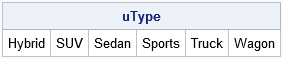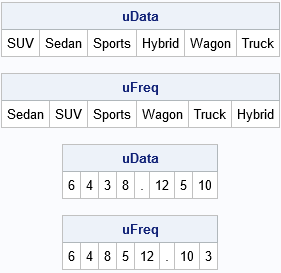There are several ways to use SAS to get the unique values for a data variable. In Base SAS, you can use the TABLES statement in PROC FREQ to generate a table of unique values (and the counts). You can also use the DISTINCT function in PROC SQL to get the same information. In the SAS/IML language, you can use the UNIQUE function to find the unique values of a vector. In all these cases, the values are returned in sorted (alphanumeric) order. For example, here are three ways to obtain the unique values of the TYPE variable in the Sashelp.Cars data set. Only the result from PROC IML is shown:

```/* Three ways to get unique values of a variable in sorted order */ proc freq data=sashelp.cars; tables Type / missing; /* MISSING option treats mising values as a valid category */ run;   proc sql; select distinct(Type) as uType from sashelp.cars; quit;   proc iml; use sashelp.cars; read all var "Type"; close;   uType = unique(Type); print uType;```### Unique values in data order

The FREQ procedure has a nice option that I use FREQently: you can use the ORDER=DATA option to obtain the unique categories in the order in which they appear in a data set. Over the years, I've used the ORDER=DATA option for many purposes, including a recent post that shows how to create a "Top 10" chart. Another useful option is ORDER=FREQ, which orders the categories in descending order by frequency.

Recently a colleague asked how to obtain the unique values of a SAS/IML vector in the order in which they appear in the vector. I thought it might also be useful to support an option to return the unique values in (descending) order of frequency, so I wrote the following function. The first argument to the UniqueOrder function is the data vector. The second (optional) argument is a string that determines the order of the unique values. If the second argument has the value "DATA", then the unique values are returned in data order. If the second argument has the value "FREQ", then the unique values are returned in descending order by frequency. The function is shown below. I test the function on a character vector (TYPE) and a numeric vector (CYLINDERS) that contains some missing values.

```proc iml; /* Return the unique values in a row vector. The second parameter determines the sort order of the result. "INTERNAL": Order by alphanumeric values (default) "DATA" : Order in which elements appear in vector "FREQ" : Order by frequency */ start UniqueOrder(x, order="INTERNAL"); if upcase(order)="DATA" then do; u = unique(x); /* unique values (sorted) */ idx = j(ncol(u),1,0); /* vector to store indices in data order */ do i = 1 to ncol(u); idx[i] = loc(x=u[i]); /* 1st location of i_th unique value */ end; call sort(idx); /* sort the indices ==> data order */ return ( T(x[idx]) ); /* put the values in data order */ end; else if upcase(order)="FREQ" then do; call tabulate(u, freq, x, "missing"); /* compute freqs; missing is valid level */ call sortndx(idx, freq`) descend=1; /* order descending by freq */ return ( T(u[idx]) ); end; else return unique(x); finish;   /* test the function by calling it on a character and numeric vectors */ use sashelp.cars; read all var "Type"; read all var "Cylinders"; close;   uData = UniqueOrder(Type, "data"); /* unique values in the order they appear in data */ uFreq = UniqueOrder(Type, "freq"); /* unique values in descending frequency order */ print uData, uFreq;   uData = UniqueOrder(Cylinders, "data"); uFreq = UniqueOrder(Cylinders, "freq"); print uData, uFreq;```The results of the function are similar to the results of PROC FREQ when you use the ORDER= option. There is a small difference when the data contain missing values. PROC FREQ always lists the missing value first, regardless of where the missing values appear in the data or how many missing values there are. In contrast, the SAS/IML function handles missing and nonmissing values equivalently.

In summary, whether you are implementing an analysis in Base SAS or SAS/IML, this article shows how to obtain the unique values of data in sorted order, in order of frequency, or in the order that the values appear in the data.

Share